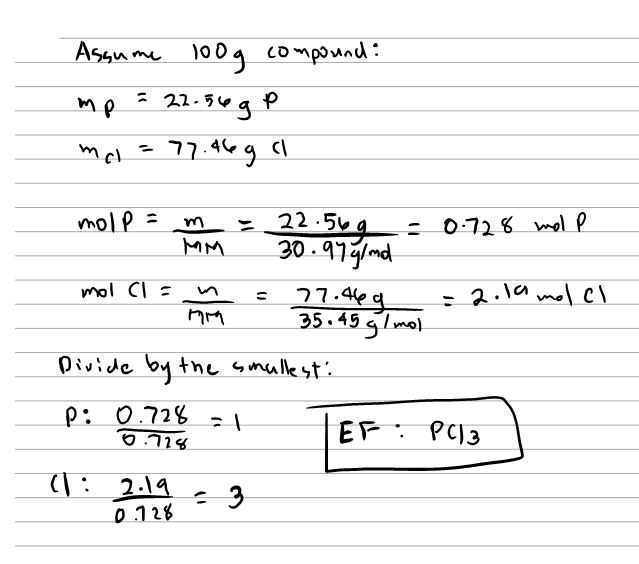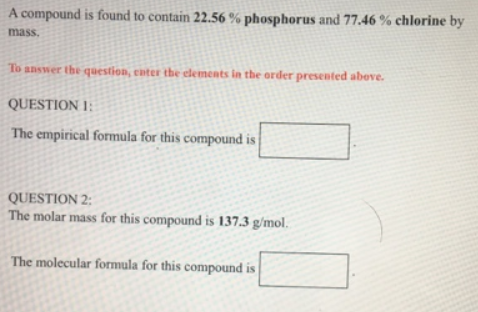# Problem: A compound is found to contain 22.56% phosphorus and 77.46% chlorine by mass. To answer the question, enter the elements in the order presented above.QUESTION 1The empirical formula for this compound is _____________ . QUESTION 2The molar mass for this compound is 137.3 g/mol. The molecular formula for this compound is _____________ .

###### FREE Expert Solution91% (5 ratings)###### Problem Details

A compound is found to contain 22.56% phosphorus and 77.46% chlorine by mass.

To answer the question, enter the elements in the order presented above.

QUESTION 1

The empirical formula for this compound is _____________ .

QUESTION 2

The molar mass for this compound is 137.3 g/mol.

The molecular formula for this compound is _____________ .